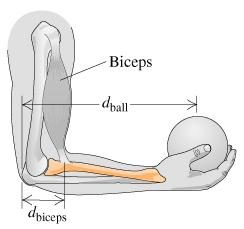# Problem: When you lift an object by moving only your forearm, the main lifting muscle in your arm is the biceps. Suppose the mass of a forearm is 1.00 kg. If the biceps is connected to the forearm a distance dbiceps = 3.50 cm from the elbow, the force Fbiceps = 113.96 N is exerted to hold a 600 g ball at the end of the forearm at distance dball = 37.0 cm from the elbow, with the forearm parallel to the floor. How much force Felbow must the elbow exert?

###### FREE Expert Solution

Weight, W = mg

For a system in equilibrium, the sum of upward forces and downward forces is equal to zero.

ΣF = 0

89% (16 ratings)###### Problem Details

When you lift an object by moving only your forearm, the main lifting muscle in your arm is the biceps. Suppose the mass of a forearm is 1.00 kg. If the biceps is connected to the forearm a distance dbiceps = 3.50 cm from the elbow, the force Fbiceps = 113.96 N is exerted to hold a 600 g ball at the end of the forearm at distance dball = 37.0 cm from the elbow, with the forearm parallel to the floor. How much force Felbow must the elbow exert?